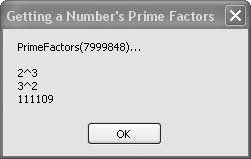# Recipe 6.22. Getting a Number s Prime Factors

### Recipe 6.22. Getting a Number's Prime Factors

#### Problem

You need to determine all prime factors of a given number, perhaps for demonstrating cryptographic algorithms or for some other purpose.

#### Solution

Sample code folder: Chapter 06\PrimeFactor

Create a function called PrimeFactors() that analyzes any Long integer and returns a string listing all the number's prime factors in a clear format.

#### Discussion

The algorithm used here is fairly straightforward, suitable for reasonably sized Long integers. The prime factors are found by checking for even divisibility by numbers from 2 to the square root of the number being checked. Whenever a factor is found, it is extracted, and the divisibility check is repeated. Tallies for the factors are converted to string format during this process, and the string is returned when all the checks are completed:

` Private Function PrimeFactors( _       ByVal numberToFactor As Long) As String    ' ----- Calculate the prime factors of a starting number.    Dim result As New System.Text.StringBuilder    Dim testFactor As Long    Dim workNumber As Long    Dim factorCount As Long    ' ----- Scan through all numbers up to    '       Sqrt(numberToFactor).    workNumber = numberToFactor    testFactor = 1    Do While (testFactor < Math.Sqrt(CType(workNumber, _          Double)))       testFactor += 1       factorCount = 0       Do While (workNumber / testFactor) = _             (workNumber \ testFactor)          ' ----- Found a factor.          factorCount += 1          workNumber \= testFactor       Loop       Select Case factorCount          Case 1             ' ----- Show a prime factor.             result.AppendLine(testFactor)          Case Is > 1             ' ----- Show a prime factor as a power.             result.Append(testFactor)             result.Append("^")             result.AppendLine(factorCount)       End Select    Loop    ' ----- Include the final prime factor, if available.    If (workNumber > 1) Then result.Append(workNumber)    Return result.ToString End Function `

Here's the code that drives the example, which finds and displays the prime factors for the number 7999848:

` Dim result As New System.Text.StringBuilder Dim number As Long = 7999848 result.AppendLine("PrimeFactors(" & number & ")… ") result.AppendLine() result.Append(PrimeFactors(number)) MsgBox(result.ToString()) `

Figure 6-22 shows the results of calculating that number's prime factors.

There are many good resources on the Web for learning about prime numbers and prime factors. See, for example, http://primes.utm.edu/largest.html.

##### Figure 6-22. Using the PrimeFactors( ) function to find all the prime factors of a number in one callVisual Basic 2005 Cookbook: Solutions for VB 2005 Programmers (Cookbooks (OReilly))
ISBN: 0596101775
EAN: 2147483647
Year: 2006
Pages: 400

Similar book on Amazon